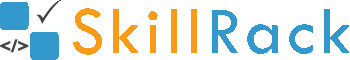Must Solve 100 Programs for Campus Placement Part - 6/104 months ago

1) Double Five Times

The program must accept an integer N as the input. The program must double the value of N for 5 times and print it as the output.

Example Input/Output 1:
Input:
5

Output:
160

Example Input/Output 2:
Input:
3

Output:
96

Click the link below to solve this program
http://www.skillrack.com/solve/8100

2) Two Digit and Three Digit

The program must accept two integers N1 and N2 as the input. The program must print yes if N1 is a two digit number and N2 is a three digit number else print no as the output.

Example Input/Output 1:
Input:
17 324

Output:
yes

Example Input/Output 2:
Input:
7 521

Output:
no

Click the link below to solve this program
http://www.skillrack.com/solve/8105

3) Same or Different - Characters

The program must accept three characters ch1, ch2 and ch3 as the input. The program must print Same if the first two characters or the last two characters are same, else print Different as the output.
Note: Print Different, if all the characters are same.

Example Input/Output 1:
Input:
s g g

Output:
Same

Example Input/Output 2:
Input:
a a a

Output:
Different

Click the link below to solve this program
http://www.skillrack.com/solve/8091

4) Sorted or Not

Accept three integers A, B and C as the input. If A, B and C are in ascending order or in descending order then print "SORTED" as the output else print "NOTSORTED" as the output.

Example Input/Output 1:
Input:
1 4 9

Output:
SORTED

Example Input/Output 2:
Input:
35 23 23

Output:
SORTED

Click the link below to solve this program
http://www.skillrack.com/solve/8095

5) Match First Three Characters

The program must accept two string values S1 and S2 as the input. The program must print YES if the first three characters are equal in S1 and S2. Else the program must print NO as the output.

Boundary Condition(s):
3 <= Length of S1, S2 <= 100

Example Input/Output 1:
Input:
manager
manhole

Output:
YES

Example Input/Output 2:
Input:
Find
finale

Output:
NO

Click the link below to solve this program
http://www.skillrack.com/solve/8096

6) Five Integers - Odd Count

Five integers are passed as the input to the program. The program must print the count of odd integers as the output.

Example Input/Output 1:
Input:
2 7 14 13 29

Output:
3

Click the link below to solve this program
http://www.skillrack.com/solve/8104

7) Absolute Difference Multiples

The program must accept two integers N1 and N2 as the input. The program must print the first 10 multiples of their absolute difference (N1 and N2) as the output.

Example Input/Output 1:
Input:
5 8

Output:
3 6 9 12 15 18 21 24 27 30

Example Input/Output 2:
Input:
7 5

Output:
2 4 6 8 10 12 14 16 18 20

Click the link below to solve this program
http://www.skillrack.com/solve/8097

8) Five Alphabets

An alphabet CH from 'A' to 'V' is passed as the input to the program. The program must print five alphabets from CH as the output. Note: The characters can be represented as integers using ASCII values.

Example Input/Output 1:
Input:
E

Output:
EFGHI

Click the link below to solve this program
http://www.skillrack.com/solve/8102

9) Same Numerator or Denominator

The program must accept two fractions as the input. Then the program must print yes as the output if the numerators of two fractions are equal or the denominators of two fractions are equal. Else the program must print no as the output.

Example Input/Output 1:
Input:
1/2 3/2

Output:
yes

Click the link below to solve this program
http://www.skillrack.com/solve/8098

10) Positive or Negative Based Multiples

Accept an integer A as the input. If A is a positive integer then print the first five multiples of the unit digit of A as the output. If A is a negative integer then print the first ten multiples of the unit digit of A as the output.

Example Input/Output 1:
Input:
46

Output:
6 12 18 24 30

Example Input/Output 2:
Input:
-53

Output:
3 6 9 12 15 18 21 24 27 30

Click the link below to solve this program
http://www.skillrack.com/solve/8107